# JavaScript Data Types

JavaScript plays a crucial role in web development, just like other programming languages do.  In JavaScript programs, you’ll encounter various data types, and this article aims to be a comprehensive guide for beginners who want to understand these “JavaScript data types

So, let us start our discussion.

## What Are Different Categories Of JavaScript Data Types?

Primarily, the data types in JavaScript can be classified into two categories. Again these two categories can be further divided into some other different types. There are eight basic data types present. We will know all about them one by one briefly.### First Category: Primitive Data Types:

Primitive data types are the most basic data types in JavaScript programming. Primitive data types are considered the very first data type of JavaScript. And such data type is developed without taking the help of other data types.

The Primitive data types are non-mutable & static. That means the value of such data type can’t flow to any other variable while the program is executing. And if any value has been provided to the variable, it can’t be changed in the program.

The Primitive data types can also be divided into some classes. There are seven primitive data types present. They are the following:

1. Number Data Type
2. String Data Type
3. BigInt Data Type
4. Boolean Data Type
5. Undefined Data Type
6. Null Data Type
7. Symbol Data Type

### Second Category: Non Primitive Data Types:

The second category we can define as the Non Primitive data type in JavaScript. The Non-primitive data types are the data types that are defined with the help of primitive data types.

Such kind of data type is Mutable in nature & dynamic also. This means the value can be easily changed during the execution of the program.

There is only one kind of Data type present in this category. It is known as the Object Data Type. We will know more about it later.

### What Is Number Data Type In JavaScript?

The Number is not at all a complex data type in JavaScript. Inside the Number, the floating point, normal numbers can be associated. Also, the exponential notation can be included in the Number category.

``````var z = 1000;  // Normal Integer Value
var a = 100.5;  // Floating Value
var p = 10e4; //  Exponential Value Evaluates To 10*10000``````

The number acts as the integer & floating values in other languages. In the case of JavaScript, there is no need to declare an Integer variable as Int & a Floating Variable as a Float. Everything here is considered a Number.

Now, the concept should become clear to you. We will now implement some programs that will help to clear the Number data type topic.

#### Play With The Number Data Type:

##### A. The Infinite & NaN Format In JavaScript:

In other languages, if we want to divide a number by zero, it gives us an error. But in the case of JavaScript, we don’t receive error messages. Rather, the Infinite message arrives.

Also, if we try to divide one string by some number, it will not give an error. Rather, it prints a NaN value that shows it is not applicable.

Code To Define Infinite & NaN Format In JavaScript:

``````const a = 10/0; // Trying To Divide Number By Zero
console.log("The Result Is: ", a); // Infinity Output

const b = "zap"/10; // Trying To Divide String By Number
console.log("The Division Result Is: ", b);  // NaN (Not Applicable) Output``````

Faced difficulties in understanding the code? no worries, you can take expert guidance for JavaScript homework help to get all your questions answered.

Output:From the output, we can see that in the first case, it is giving the Infinity message. And in the second case, it is giving the message NaN. So, the implementation of the program is errorless.

Now, let us look at another example of using Numbers.

#### B. Dynamic Nature Of Numbers:

The number of variables can become dynamic. If the variable has earlier assigned to one number data type & now it is assigned with the String value. The same variable will be going to point to the string value.

Here, one variable earlier had a number value. We have changed the value to a string. Now, if we try to print the variable, we will get the string value, not the number value.

Code To Define Dynamic Nature Of Numbers In JavaScript:

``````let x; // Declaration Of Variable
x = 5; // Variable Is Now A Number
x = "ZapOne"; // Variable Is Now A String

console.log("The New Value Is: ", x); // Printing The Value``````

Output:The output clearly shows that the variable has now the value of the string. It has removed the value of the number that it has earlier.

### What Is String Datatype In JavaScript?

In JavaScript, the string values are defined as the Textual Data. That means, all the textual formats can be described as the String in JavaScript. And the String concept is the same just like other languages.

``````var s1 = “Double Quote Sting”;
var s2= ‘Single Quote Sting’;
var s3 = `Tilda Sting`;``````

In JavaScript, the strings are declared with the help of Single or Double Quotes. Sometimes, the Tilda symbol is also used for the declaration process.

We can implement some of the programs to get a clear understanding of Strings.

#### Play With The Strings In JavaScript:

##### A. Game Of Single or Double Quotes:

In JavaScript strings, the use of single & double quotes can also be possible inside any string. To demark, any string, we should use the Single or Double quotes.

But what if, we need to use another set of single & double quotes inside of the string?

Code To Define Use Of Quotes Inside Of Stirngs In JavaScript:

``````let a = "-It Is 'ZapOne'-"; // Single Quote Inside Double Quote String
let b = '-It Is "CodingZap"-'; // Double Quote Inside Inside Quote String

console.log("The First String Is: ", a); // Printing First One
console.log("The Second String Is: ", b); // Printing Second One``````

Output: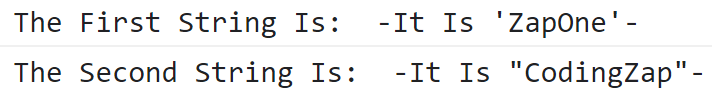From the above output, we can see that we have successfully used the single quote inside the double quote string. And in the second case, the double quotes are successfully used inside of the single quote string.

##### B. Concatenation Of Strings & Numbers:

The concatenation process of the strings is very interesting in JavaScript. If we are trying to concatenate a string along with one number, so every time the number will not be considered as a string. It depends upon the placement process.

If only two values are pasted at the beginning of the concatenation process, it will act as the number. But if there is only one value present, it will be considered a string. But if values are pasted at the end, they will be treated as strings every time irrespective of their numbers.

Code To Demonstrate Concatenation Of Strings & Numbers In JavaScript:

``````let a1 = 10 + 20 + "ZapOne"; // Numbers Are Pasted In The Front
let a2 = "CodingZap" + 10 + 20; // Numbers Are Pasted At The End

console.log("The First Result Is: ", a1); // Printing First One
console.log("The Second Result Is: ", a2); // Printing Second One``````

Output:In the above output, in the case of the first result, the numbers are added before going for concatenation as they are present in the front. In the second case, the numbers don’t add to each other. Rather, they are all concatenated as they are present at the backside.

### What Is BigInt Data Type In JavaScript?

BigInt is another important concept in the JavaScript program. The BigInt is necessary to work with big integer values. In the case of the BigInt, the numbers are not considered floating point numbers.

``````let a = BigInt("978456789044444678901234567890"); // Declaration With BigInt()
const value1 = 500071555554740998n; // Declaration With 'n' At The End``````

Code To Demonstrate BigInt Data Type In JavaScript:

``````const e1 = 500071555554740998n; // Declaration Of Big Value
const r1 = e1 + 2n; // Adding With Another Big Value
console.log("The Result Value Will Be: ", r1); // Printing The Value``````

Output: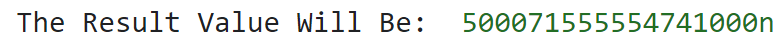From the above output, we can see that the BigInt value is added with another bog value. And the complete value is printed in the program without using any floating point or decimal point values.

### What Is Boolean Value In JavaScript?

The Boolean values in the JavaScript program act as the same in other languages. The Boolean values consist of only two types of values. One is the Boolean True value & another is the Boolean False value.

``````const a = true; // True Boolean Value
const b = false; // False Boolean Data``````

Code To Define Boolean Values In JavaScript:

``````let d = 10; // First Value
let f = 10; // Second Value
let v = 22; // Third Value

console.log ("The Boolean Value In First Two Variables Is: ", (d == f));
console.log ("The Boolean Value In Last Two Variables Is: ", (f == v));``````

Output: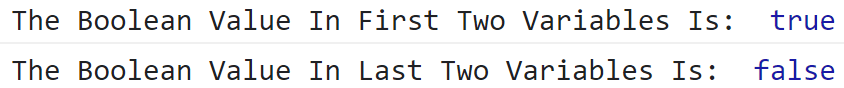As the first condition is satisfying, the respective result is giving the Boolean True Value. And in the next case, the condition is not satisfying with the data. So, the result is coming as Boolean False.

### What Is Undefined Data Type In JavaScript?

Undefined data type means whatever is declared as the variable in the program is not initiated at all. If any value has been given to the variable, it will not be considered as the Undefined element.

Code To Check Syntax & Use Of Undefined Operation:

``````let x; // Declaring Variable
console.log("The Value Is: ", x); // Printing Without Value Assigning``````

In this case, the variable is not defined with values. And we are trying to print the value. So, it will give the Undefined message as the output.

### What Is Null Value In JavaScript?

The Null value occurs in JavaScript, when we are providing the specific Null data to some variable. In such a case, if we are trying to print the variable, it will not give us the Undefined message. Because the value is changed to Null type.

Code To Check Syntax & Use Of Null Operation:

``````let x = null; // Declaring Variable With Null
console.log("The Value Is: ", x); // Printing Value``````

In the above case, we have provided the Null Value to the variable. Now, if we try to print the value. We will get the Null message rather than Undefined. That means the variable is declared successfully.

### What Is Symbol Data Type In JavaScript?

The Symbol is the newest ever element that is added to the JavaScript programs. In 2015, it was first introduced. The Symbol Data Type can be worked with the help of the Object Data Type. The declaration can be done with the Symbol() function data type.

Code To Define Symbol Element In JavaScript:

``````const b1 = Symbol('ZapOne'); // First Symbol
const b2 = Symbol('CodingZap'); // Second Symbol

console.log("First Initiated Symbol: ", b1); // Printing First Symbol
console.log("Second Initiated Symbol: " ,b2); // Printing Second Symbol``````

Output: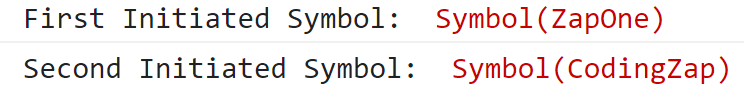From the output, we can see that the two symbols are declared successfully. The Symbol “CodingZap” & Symbol “ZapOne” will be used as the tags to make some other components inside the program.

### What Is Object Data Type In JavaScript?

JavaScript object element has some similarities with the Structure Data Structures concept. The Object element developed with the help of other primary elements that we discussed earlier. The Object is defined with the Key value pairs.

``````const code = {
Name: 'JavaScript',
Popularity: 5
};``````

One of the examples is declared above. We have introduced some key-value pairs in order to understand the concept. The Object is itself a complete section. That needs to be discussed in a separate format. Because the object is the gateway of advanced topics in JavaScript.

#### Bonus Note: What Is Typeof() Operator In JavaScript?

Typeof operator is an essential element present in the JavaScript program that helps to find out the type of data element used in any program. We have to use the variable name inside that typeof operator.

If you are not able to understand what element is used, you can use the typeof() operator function. Though, the chances of that are very rare because all the parts have been discussed well form.

``General Syntax: typeof(variable-name);``

Code To Demonstrate The Typeof() Operator:

``````const t = 'ZapOne'; // First Variable
console.log("The Type Is: ", typeof(t)); // Returns "string" Type

const u = 2023; // Second Variable
console.log("The Type Is: ", typeof(u)); // Returns "number" Type``````

Output: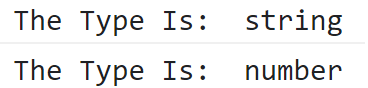In the above output, we can see, in the first case, it is giving type as String because not a number is used there. In the second case, one integer value is used. So, the Number type is arriving.

## Conclusion:

These are some important datatype that belongs to the JavaScript program.

The concept of these variables & datatypes should be clear to you because it is going to be a gateway for your journey toward the Web Development process. All the Data Structures should be cleared. Still. if you wish to learn how JavaSciprt in Web Development works, you can check out our article.

In this article, we have used a few more programs that help to clear the concept of that data. We recommend you practice the concept as much as possible. Because practice is the only key to grabbing knowledge easily.